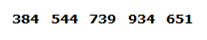# RRB PO Prelims Reasoning Ability Questions 2021 (Day-04)

Dear Aspirants, Our IBPS Guide team is providing new series of Reasoning Questions for RRB PO Prelims 2021 so the aspirants can practice it on a daily basis. These questions are framed by our skilled experts after understanding your needs thoroughly. Aspirants can practice these new series questions daily to familiarize with the exact exam pattern and make your preparation effective.

Start Quiz

Designation puzzle

Direction (1-5): Study the following information carefully and answer the below questions.

There are eight employees of the educational institute named M, N, O, P, Q, R, S, and T. They have different designations starting from Clerk, Head Clerk, Assistant Professor, Professor, HOD, Supervisor, Principal, and Director. All the designations given are to be considered in a given order (as Clerk is considered as Junior-most rank and Director is considered as the Senior-most rank).

Only two persons are junior rank to O. S is three ranks senior to Q. Q’s rank is neither immediate above nor immediate below to O. R is immediate senior rank to M. P is a Head Clerk. N is two ranks junior to M. T is not a Supervisor.

1) Which of the following statement is not true?

A.R is a Principal.

B.The one who is Supervisor is immediate senior rank to Q.

C.The one who is Assistant Professor is immediate junior rank to N.

D.Q is a Clerk.

E.All are true.

2) Who is the immediate junior rank to the one who is Professor?

A.R

B.Q

C.O

D.S

E.None of these

3) Which of the following statement is/are true?

A.S is Director.

B.M is immediate senior rank to HOD.

C.Only two persons are senior rank than Q.

D.Both a and b

E.Both b and c

4) Four of the following five pairs of designations and employees have a certain relationship with each other. Which one does not belong to the group?

A.S- Supervisor

B.M- HOD

C.Q- Assistant Professor

D.O- Clerk

5) Who among the following persons are junior-most and senior-most employees respectively?

A.T, S

B.Q, R

C.S, T

D.R, Q

E.None of these

Number series

Direction (6-10): Study the following information carefully and answer the below questions.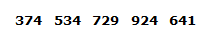6) If all the digits of the number are written in ascending order within the number, then which of the following will be the sum of the middle digits of all the numbers?

A.11

B.38

C.23

D.27

E.29

7) If all the digits of the number are written in descending order within the number, then which of the following will be the second-lowest number?

A.534

B.641

C.729

D.374

E.924

8) If the 3rd digit of the 2nd highest number is divided by the 2nd digit of the 2nd lowest number, then what will be the resultant?

A.1

B.9

C.6

D.3

E.None of these

9) If we add the 1st and 3rd digits of each number, then which of the following will be the third-highest number?

A.924

B.729

C.534

D.641

E.374

10) If 1 is added to the second digit of each number then how many numbers thus formed will be divisible by 3?

A.Three

B.Two

C.One

D.Four

E.None

Directions (1-5) :

There are eight employees of the educational institute named M, N, O, P, Q, R, S, and T. They have different designations starting from Clerk, Head Clerk, Assistant Professor, Professor, HOD, Supervisor, Principal, and Director. Clerk is considered as junior-most rank and Director is considered as senior-most rank.

Only two persons are junior rank to O. S is three ranks senior to Q. Q’s rank is neither immediate above nor immediate below to O. R is immediate senior rank to M. P is a Head Clerk. N is two ranks junior to M.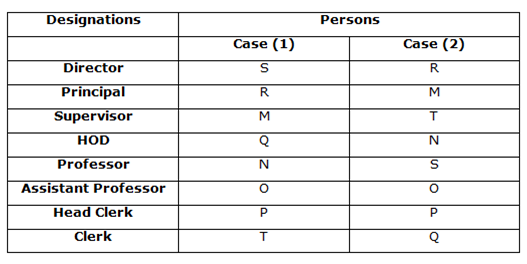T is not a Supervisor.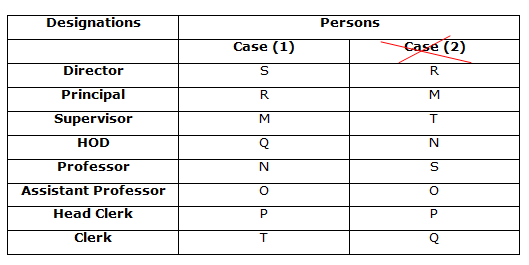Case (1) is the final answer.

all the digits of the number are written in ascending order within the number, then we will get the below sequence.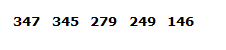Sum of the middle digits of all the numbers = 4 + 4 + 7 + 4 + 4 = 23

all the digits of the number are written in descending order within the number, then we will get the below sequence.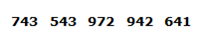The second-lowest number is 641.

924 >72> 641 > 534 > 374

3rd digit of 2nd highest number = 9

2nd digit of 2nd lowest number = 3

So, 9/3 = 3

374=> 3+4 = 7

534=> 5+4 = 9

729=> 7+9 = 16

924=> 9+4 = 13

641=> 6+1 = 7